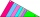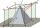# Steps

Find the height between the two floors if you know that the number of steps between the two floors is 18, the gradient is 30º, and the length of the step is 28.6 cm. Report the result in centimeters to the nearest centimeter.

x =  297 cm

### Step-by-step explanation:Did you find an error or inaccuracy? Feel free to write us. Thank you!Tips to related online calculators
Do you want to convert length units?

#### You need to know the following knowledge to solve this word math problem:

We encourage you to watch this tutorial video on this math problem:

## Related math problems and questions:

• Right triangleCalculate the length of the remaining two sides and the angles in the rectangular triangle ABC if a = 10 cm, angle alpha = 18°40'.
• Right triangle trigonometricsCalculate the size of the remaining sides and angles of a right triangle ABC if it is given: b = 10 cm; c = 20 cm; angle alpha = 60° and the angle beta = 30° (use the Pythagorean theorem and functions sine, cosine, tangent, cotangent)
• Sin cos tanIn triangle ABC, right-angled at B. Sides/AB/=7cm, /BC/=5cm, /AC/=8.6cm. Find to two decimal places. A. Sine C B. Cosine C C. Tangent C.
• StairwayWhat angle rising stairway if step height in 20 cm and width 26 cm?The height of the regular quadrangular pyramid is 6 cm, the length of the base is 4 cm. What is the angle between the ABV and BCV planes?
• RectangleCalculate the length of the side GN and diagonal QN of rectangle QGNH when given: |HN| = 25 cm and angle ∠ QGH = 28 degrees.
• Right angleIn a right triangle ABC with a right angle at the apex C, we know the side length AB = 24 cm and the angle at the vertex B = 71°. Calculate the length of the legs of the triangle.
• Space diagonal anglesCalculate the angle between the body diagonal and the side edge c of the block with dimensions: a = 28cm, b = 45cm and c = 73cm. Then, find the angle between the body diagonal and the plane of the base ABCD.
• Right triangleA right triangle ABC is given, c is a hypotenuse. Find the length of the sides a, b, the angle beta if c = 5 and angle alfa = A = 35 degrees.
• TrapezoidsIn the isosceles trapezoid ABCD we know: AB||CD, |CD| = c = 8 cm, height h = 7 cm, |∠CAB| = 35°. Find the area of the trapezoid.
• Power line poleFrom point A, the power line pole is seen at an angle of 18 degrees. From point B to which we get when going from point A 30m away from the column at an angle of 10 degrees. Find the height of the power pole.
• Parallelogram +ľ| AB | = 76cm, | BC | = 44cm, angle BAD = 30 °. Find the area of the parallelogram.
• IS triangleCalculate interior angles of the isosceles triangle with base 40 cm and legs 22 cm long.
• ParallelogramFind the parallelogram's perimeter, where base a = 8 cm, height v = 3 cm, and angle alpha = 35° is the magnitude of the angle at vertex A.
• A trapezoidA trapezoid with a base length of a = 36.6 cm, with angles α = 60°, β = 48° and the height of the trapezoid is 20 cm. Calculate the lengths of the other sides of the trapezoid.
• BoxCalculate the angle between box base 9 x 14 and body diagonal length 18.
• Cuboid diagonalCalculate the volume and surface area of the cuboid ABCDEFGH, which sides a, b, c has dimensions in the ratio of 9:3:8. If you know that the diagonal wall AC is 86 cm, and the angle between AC and space diagonal AG is 25 degrees.